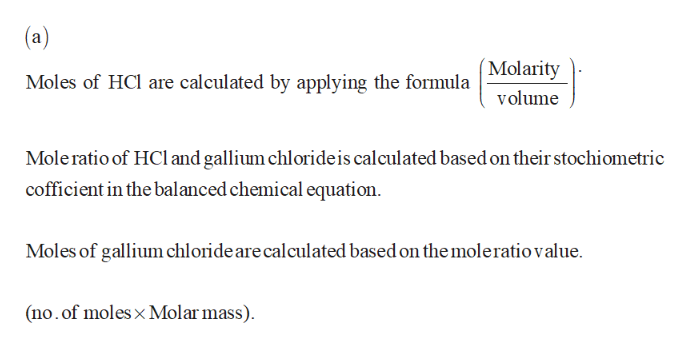# Gallium chloride is formed by the reaction of 2.6 L of a 1.44 M solution of HCl according to the following equation: 2Ga+6HCl ⟶ 2GaCl3+3H2.(a) Outline the steps necessary to determine the number of moles and mass of gallium chloride.(b) Perform the calculations outlined.

Question
231 views

Gallium chloride is formed by the reaction of 2.6 L of a 1.44 M solution of HCl according to the following equation: 2Ga+6HCl ⟶ 2GaCl3+3H2.

(a) Outline the steps necessary to determine the number of moles and mass of gallium chloride.

(b) Perform the calculations outlined.

check_circle

Step 1help_outlineImage Transcriptionclosea Molarity Moles of HCl are calculated by applying the formula volume Mole ratio of HCl and gallium chloride is calculated based on their stochiometric cofficient in the balanced chemical equation Moles of gallium chloride are calculated based on the moleratio value (no.of moles x Molar mass) fullscreen
Step 2

(b)

Volume of HCl solution = 2.6 L

Molarity of HCl solution ...

### Want to see the full answer?

See Solution

#### Want to see this answer and more?

Solutions are written by subject experts who are available 24/7. Questions are typically answered within 1 hour.*

See Solution
*Response times may vary by subject and question.
Tagged in

### Chemistry